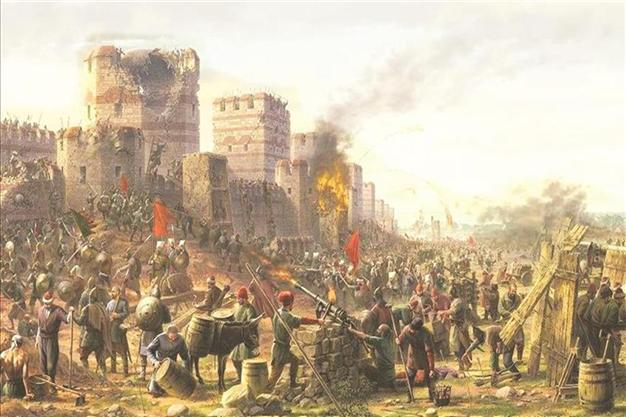# Cannon Shot With Air ResistanceYou're a $15^\text{th}$ century ballistics expert serving under the great Ottoman general Sultan Mehmed the Conqueror, tasked with ensuring a solid connection between cannon fire and the heavily fortified city walls of Constantinople.

You're setting up the cannon, which has a muzzle velocity of $v_0 = \SI[per-mode=symbol]{300}{\meter\per\second},$ for a shot at a crucial encampment some $\SI{2000}{\meter}$ away at the heart of the city when a few upstarts in your outfit start yelling at you, upset that you insist upon careful evaluation of the flight path of the cannonball, and urge you to take a more freewheeling approach. Historians will later note a band of Ottomans screaming at a dejected engineer, "just let it rip."

You hold steady and tell them to find something else to do.

Suppose you allowed the yahoos to have their way and ignored the effects of wind drag. By what margin $($in $\si{\meter})$ would your cannon fire undershoot the encampment?

Assume that the force of wind resistance has the form $\mathbf{F}_\textrm{drag} = -\frac12 \rho_\textrm{air}C_d Av^2 \hat{\mathbf{v}},$ where $\hat{\mathbf{v}}$ is a unit vector in the direction of the total velocity $\mathbf{v},$ $A$ is the cross-sectional area of the cannonball, and the constants are as defined in the code box below (in SI units). Moreover, the cannonball is a steel sphere of radius $r$ and density $\rho_\textrm{steel}.$ To avoid collateral damage, you only choose launch angles $\theta \geq \SI{40}{\degree}.$ For historical context, read on the Fall of Constantinople.

import math

rho_air = 1.22
g = 10.0
rho_steel = 8050.0
v0 = 300.0
r = 0.08
theta = # Fill this in.
drag = "Off" # Set this to "On"  or "Off" to include drag, or not.
C_d = (1 if drag is "On" else 0)

(vx, vy) = (v0 * math.cos(theta * math.pi / 180), v0 * math.sin(theta * math.pi / 180))
(x, y) = (0, 0)

dt = 0.001

while y >= 0:
# Finish this code to step through the motion of the cannonball.
(x, y) = (x + ..., y + ...)
(vx, vy) = (vx + ..., vy + ...)

print("The cannonball's range is {:.2f} meters.".format(x))

Python 3
You need to be connected to run code

×